#### Autocorrelation Statistics Types

One measure of spatial autocorrelation provided by PROC VARIOGRAM is Moran’s I statistic, which was introduced by Moran (1950) and is defined as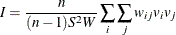where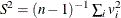, and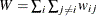.

Another measure of spatial autocorrelation in PROC VARIOGRAM is Geary’s c statistic (Geary, 1954), defined as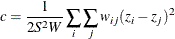These expressions indicate that Moran’s I coefficient makes use of the centered variable, whereas the Geary’s c expression uses the noncentered values in the summation.

Inference on these two statistic types comes from approximate tests based on the asymptotic distribution of I and c, which both tend to a normal distribution as n increases. To this end, PROC VARIOGRAM calculates the means and variances of I and c. The outcome depends on the assumption made regarding the distribution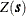. In particular, you can choose to investigate any of the statistics under the normality (also known as Gaussianity) or the randomization assumption. Cliff and Ord (1981) provided the equations for the means and variances of the I and c distributions, as described in the following.

The normality assumption asserts that the random fieldfollows a normal distribution of constant mean (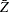) and variance, from which the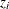values are drawn. In this case, the I statistics yield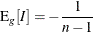andwhere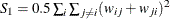and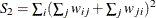. The corresponding moments for the c statistics are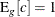and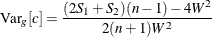According to the randomization assumption, the I and c observations are considered in relation to all the different values that I and c could take, respectively, if the nvalues were repeatedly randomly permuted around the domain D. The moments for the I statistics are now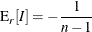and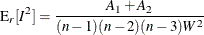where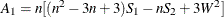,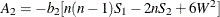. The factor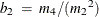is the coefficient of kurtosis that uses the sample moments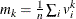for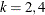. Finally, the c statistics under the randomization assumption are given by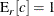and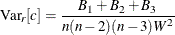with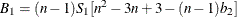,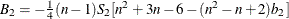, and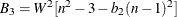.

If you specify LAGDISTANCE= to be larger than the maximum data distance in your domain, the binary weighting scheme used by the VARIOGRAM procedure leads to all weights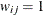,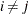. In this extreme case the preceding definitions can show that the variances of the I and c statistics become zero under either the normality or the randomization assumption.

A similar effect might occur when you have collocated observations (see the section Pair Formation). The Moran’s I and Geary’s c statistics allow for the inclusion of such pairs in the computations. Hence, contrary to the semivariance analysis, PROC VARIOGRAM does not exclude pairs of collocated data from the autocorrelation statistics.